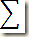# ieCivil

Engineering the Digital Civilization

## Tuesday, 18 August 2015

Assalamu’Alaykum,

• The calculator Casio fx-991MS having too many facilities that can aid students in hard times. For the particular instance, the regression mode.
• Many a times in many syllabus Statistics is included which is very scoring and easy but the problem is it consumes a lot of time and if not proceeded cautiously a single mistake makes the entire solution wrong.

Consider the example of the following data table.

 x 1 3 5 7 9 11 13 17 19 23 y 2 4 6 8 10 17 51 62 7 15
here we’re asked to compute mean, variance, standard deviation, cov(x,y), and co-efficient of co-variance ‘r’.
The conventional method that is used by the students all across is to draw a table and then calculate various values like
And for the current example a table as follows is made

 n x y x2 y2 xy 1 1 2 2 3 4 3 5 6 4 7 8 5 9 10 6 11 17 7 13 51 8 17 62 9 19 7 10 23 15and then the values under the headings are calculated as per the formula. Then all the values are added.

## Short-Cut

Using the calculator the desired quantity can be calculated within one or two steps

1. : Turn on the calculator
2. : Get into the regression mode by pressing 2
3. : Select the linear mode by pressing 1
4. : Congratulations! You’re now in the regression mode
5. : Feed the values of x and y by pressing the values and separating them ',' (comma) and then press the M+ button to save the value in the calculator.
6. Save all the values using the above steps, and when you're done press AC button.
7. Now to calculate the mean of x press “ Shift” then press “2” and then press “1”.
8. Now to calculate the mean of y press “ Shift” then press “2” then press “right navigation” and then press “1”.
9. Now to calculate variance of x press “ Shift” then press “2” and then press “2”.
10. Now to calculate variance of x press “ Shift” then press “2” then press “right navigation” and then press “2”.
11. Now to calculate the co-relation coefficient ‘r’ press “ Shift” then press “2” then press “right navigation” then press “right navigation” and then press “3”.

Thus saving a lot of time and labour with optimum accuracy….

A video demonstrating the above steps in detail will be posted in some days…